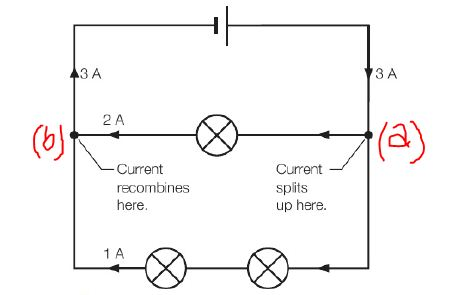# How current flows through series and parallel circuits? A quick reference

Last updated on April 14th, 2021 at 04:33 pm

Here we will see how current flows through series and parallel circuits.

## Currents in series circuits

In a series circuit, devices are connected so there is only one closed path for current to follow. However, if any part of this path is broken, current will no longer flow in the circuit.

Any group of components that are connected in series in a circuit, with no junctions in the circuit, must have the same current through them all.

## Currents in parallel circuits

In a parallel circuit, devices are connected so there is more than one closed path for current to follow. If the current flow is broken in one path, current will continue to flow in the other paths.

Whenever a current encounters a junction in a circuit (parallel circuit), the charges have more than one path to flow. So the current must split. The proportions that travel along each possible path will be in inverse proportion to the resistance along that path. If the path has high resistance, the current flow will be less through that path and vice versa. Finally, the total current along the parallel branches must add up to the original total current, in order to conserve charge.Current splits at circuit junction (see point a in the figure) and recombines (at point b).

Similar topics

Equivalent resistance in series circuit

Equivalent resistance in a parallel circuit